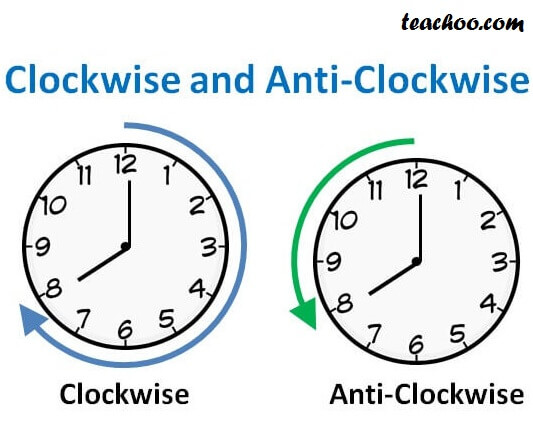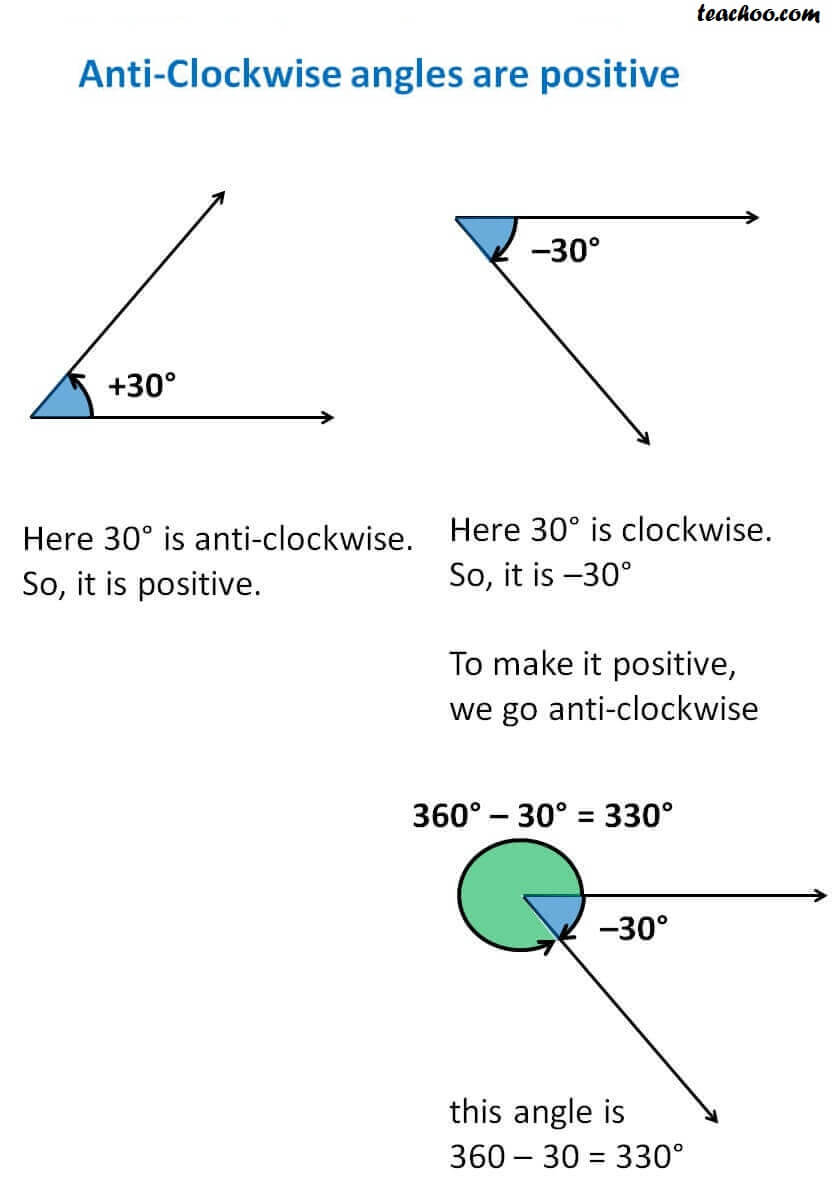Chapter 3 Class 11 Trigonometric Functions
Concept wise

First we will study what is clockwise and anti-clockwise

## What is clockwise and anti-clockwise?

Clockwise means moving in direction of clock.

Anti-clockwise means moving in opposite direction of clock## Anti-Clockwise angles are positiveIn the left, 30° is anti-clockwise.

So, it is positive.

In the right, Here 30° is clockwise.

So, it is –30°

In positive,

this angle is

360 – 30 = 330°

Learn in your speed, with individual attention - Teachoo Maths 1-on-1 Class

### Transcript

First we will study what is clockwise and anti-clockwise What is clockwise and anti-clockwise? Clockwise means moving in direction of clock. Anti-clockwise means moving in opposite direction of clock Anti-Clockwise angles are positive In the left, 30° is anti-clockwise. So, it is positive. In the right, Here 30° is clockwise. So, it is –30° In positive, this angle is 360 – 30 = 330°